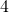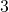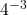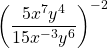# Simplify Expressions with Exponents

The purpose of the exercise in this lesson is to develop some muscle memory with exponent laws, which helps avoid to errors in future work and develop some efficiencies.

Students studying remotely: Please print the worksheet linked at the end of the page. Choose 5 questions from each column in the exercise. Show your steps for simplifying each expression. Check the answers to see if you have made correct sense of each expression.

## Example 1

SimplifyIt is important to remember that the exponent applies to only what it is written beside. The exponentapplies to the, not to the. Written out in full we have:In short,## Example 2

SimplifyThe exponent 3 is applied to each factor in the bracket:## Example 3

SimplifyA negative exponent means to divide repeatedly. We ‘take the reciprocal‘. The result here is a small, positive number.## Example 4

SimplifyIt is helpful here to create a new law:

SimplifyIn short,Applying this new law, we haveand.## Example 5

SimplifyIt is helpful to gather like terms as follows:## Example 6

SimplifyLet’s make another new law.

SimplifyIn short,Apply this new law to example 6 we haveWorksheet